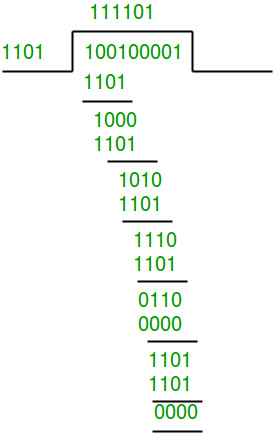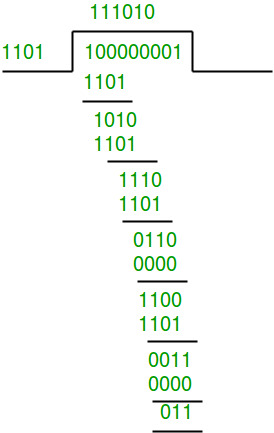# Cyclic Redundancy Check and Modulo-2 Division

CRC or Cyclic Redundancy Check is a method of detecting accidental changes/errors in communication channel.

CRC uses Generator Polynomial which is available on both sender and receiver side. An example generator polynomial is of the form like x3 + x + 1. This generator polynomial represents key 1011. Another example is x2 + 1 that represents key 101.

```n : Number of bits in data to be sent
from sender side.
k : Number of bits in the key obtained
from generator polynomial. ```

Sender Side (Generation of Encoded Data from Data and Generator Polynomial (or Key)):

1. The binary data is first augmented by adding k-1 zeros in the end of the data
2. Use modulo-2 binary division to divide binary data by the key and store remainder of division.
3. Append the remainder at the end of the data to form the encoded data and send the same
4. .

Receiver Side (Check if there are errors introduced in transmission)
Perform modulo-2 division again and if remainder is 0, then there are no errors.

In this article we will focus only on finding the remainder i.e. check word and the code word.

Modulo 2 Division:
The process of modulo-2 binary division is the same as the familiar division process we use for decimal numbers. Just that instead of subtraction, we use XOR here.

• In each step, a copy of the divisor (or data) is XORed with the k bits of the dividend (or key).
• The result of the XOR operation (remainder) is (n-1) bits, which is used for the next step after 1 extra bit is pulled down to make it n bits long.
• When there are no bits left to pull down, we have a result. The (n-1)-bit remainder which is appended at the sender side.

Illustration:

Example 1 (No error in transmission):

```Data word to be sent - 100100
Key - 1101 [ Or generator polynomial x3 + x2 + 1]

Sender Side:Therefore, the remainder is 001 and hence the encoded
data sent is 100100001.Therefore, the remainder is all zeros. Hence, the
data received has no error. ```

Example 2: (Error in transmission)

```Data word to be sent - 100100
Key - 1101

Sender Side:Therefore, the remainder is 001 and hence the
code word sent is 100100001.

Let there be error in transmission mediaSince the remainder is not all zeroes, the error
is detected at the receiver side.```

Implementation

Below is Python implementation for generating code word from given binary data and key.

 `# Returns XOR of 'a' and 'b' ` `# (both of same length) ` `def` `xor(a, b): ` ` `  `    ``# initialize result ` `    ``result ``=` `[] ` ` `  `    ``# Traverse all bits, if bits are ` `    ``# same, then XOR is 0, else 1 ` `    ``for` `i ``in` `range``(``1``, ``len``(b)): ` `        ``if` `a[i] ``=``=` `b[i]: ` `            ``result.append(``'0'``) ` `        ``else``: ` `            ``result.append(``'1'``) ` ` `  `    ``return` `''.join(result) ` ` `  ` `  `# Performs Modulo-2 division ` `def` `mod2div(divident, divisor): ` ` `  `    ``# Number of bits to be XORed at a time. ` `    ``pick ``=` `len``(divisor) ` ` `  `    ``# Slicing the divident to appropriate ` `    ``# length for particular step ` `    ``tmp ``=` `divident[``0` `: pick] ` ` `  `    ``while` `pick < ``len``(divident): ` ` `  `        ``if` `tmp[``0``] ``=``=` `'1'``: ` ` `  `            ``# replace the divident by the result ` `            ``# of XOR and pull 1 bit down ` `            ``tmp ``=` `xor(divisor, tmp) ``+` `divident[pick] ` ` `  `        ``else``:   ``# If leftmost bit is '0' ` `            ``# If the leftmost bit of the dividend (or the ` `            ``# part used in each step) is 0, the step cannot ` `            ``# use the regular divisor; we need to use an ` `            ``# all-0s divisor. ` `            ``tmp ``=` `xor(``'0'``*``pick, tmp) ``+` `divident[pick] ` ` `  `        ``# increment pick to move further ` `        ``pick ``+``=` `1` ` `  `    ``# For the last n bits, we have to carry it out ` `    ``# normally as increased value of pick will cause ` `    ``# Index Out of Bounds. ` `    ``if` `tmp[``0``] ``=``=` `'1'``: ` `        ``tmp ``=` `xor(divisor, tmp) ` `    ``else``: ` `        ``tmp ``=` `xor(``'0'``*``pick, tmp) ` ` `  `    ``checkword ``=` `tmp ` `    ``return` `checkword ` ` `  `# Function used at the sender side to encode ` `# data by appending remainder of modular divison ` `# at the end of data. ` `def` `encodeData(data, key): ` ` `  `    ``l_key ``=` `len``(key) ` ` `  `    ``# Appends n-1 zeroes at end of data ` `    ``appended_data ``=` `data ``+` `'0'``*``(l_key``-``1``) ` `    ``remainder ``=` `mod2div(appended_data, key) ` ` `  `    ``# Append remainder in the original data ` `    ``codeword ``=` `data ``+` `remainder ` `    ``print``(``"Remainder : "``, remainder) ` `    ``print``(``"Encoded Data (Data + Remainder) : "``, ` `          ``codeword) ` ` `  `# Driver code ` `data ``=` `"100100"` `key ``=` `"1101"` `encodeData(data, key) `

Output:

```Remainder :  001
Encoded Data (Data + Remainder) :  100100001
```

Note that CRC is mainly designed and used to protect against common of errors on communication channels and NOT suitable protection against intentional alteration of data (See reasons here)

This article is contributed by Jay Patel. If you like GeeksforGeeks and would like to contribute, you can also write an article and mail your article to contribute@geeksforgeeks.org. See your article appearing on the GeeksforGeeks main page and help other Geeks.